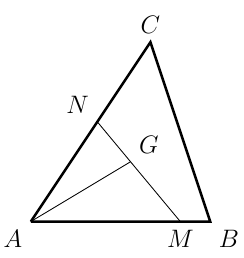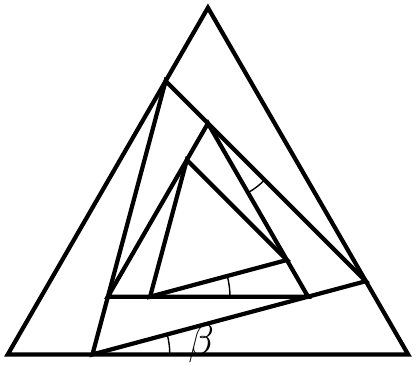###### back to index | new

Let even function $f(x)$ and odd function $g(x)$ satisfy the relationship of $f(x)+g(x)=\sqrt{1+x+x^2}$. Find $f(3)$.

Let $f\Big(\dfrac{1}{x}\Big)=\dfrac{1}{x^2+1}$. Compute $$f\Big(\dfrac{1}{2013}\Big)+f\Big(\dfrac{1}{2012}\Big)+f\Big(\dfrac{1}{2011}\Big)+\cdots +f\Big(\dfrac{1}{2}\Big)+f(1)+f(2)+\cdots +f(2011)+f(2012)+f(2013)$$

Let $f(x)=x^{-\frac{k^2}{2}+\frac{3}{2}k+1}$ be an odd function where $k$ is an integer. If $f(x)$ is monotonically increasing when $x\in(0,+\infty)$, find all the possible values of $k$.

Let $G$ be the centroid of $\triangle{ABC}$. Points $M$ and $N$ are on side $AB$ and $AC$, respectively such that $\overline{AM} = m\cdot\overline{AB}$ and $\overline{AN} = n\cdot\overline{AC}$ where $m$ and $n$ are two positive real numbers. Find the minimal value of $mn$.An infinite number of equilateral triangles are constructed as shown on the right. Each inner triangle is inscribed in its immediate outsider and is shifted by a constant angle $\beta$. If the area of the biggest triangle equals to the sum of areas of all the other triangles, find the value of $\beta$ in terms of degrees.Suppose function $f(x)=\frac{1+x}{1-x}$. Evaluate $$f\Big(\frac{1}{2}\Big)\cdot f\Big(\frac{1}{4}\Big)\cdot f\Big(\frac{1}{6}\Big)\cdots f\Big(\frac{1}{2014}\Big)$$

Let the sum of first $n$ terms of arithmetic sequence $\{a_n\}$ be $S_n$, and the sum of first $n$ terms of arithmetic sequence $\{b_n\}$ be $T_n$. If $\frac{S_n}{T_n}=\frac{2n}{3n+7}$, compute the value of $\frac{a_8}{b_6}$.

Suppose every term in the sequence $$1, 2, 1, 2, 2, 2, 1, 2, 2, 2, 2, 2, 1, \cdots$$ is either $1$ or $2$. If there are exactly $(2k-1)$ twos between the $k^{th}$ one and the $(k+1)^{th}$ one, find the sum of its first $2014$ terms.

Let $c_1, c_2, c_3, \cdots$ be a series of concentric circles whose radii form a geometric sequence with common ratio as $r$. Suppose the areas of rings which are formed by two adjacent circles are $S_1, S_2, S_3, \cdots$. Which statement below is correct regarding the sequence $\{S_n\}$? A) It is not a geometric sequence B) It is a geometric sequence and its common ratio is $r$ C) It is a geometric sequence and its common ratio is $r^2$ D) It is a geometric sequence and its common ratio is $r^2-1$

Given the sequence $\{a_n\}$ satisfies $a_n+a_m=a_{n+m}$ for any positive integers $n$ and $m$. Suppose $a_1=\frac{1}{2013}$. Find the sum of its first $2013$ terms.

If real number $x$ satisfies $x^4 - 2x^3 -7x^2 + 8x +12\le 0$, find the max value of $|x+\frac{4}{x}|$

Find the range of function $f(x)=3^{-|\log_2x|}-4|x-1|$.